Foxtable(狐表)用户栏目专家坐堂 → [求助]客户编号

共有1827人关注过本帖树形打印复制链接

主题：[求助]客户编号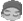1楼 | 信息 | 搜索 | 邮箱 | 主页 | UC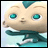加好友发短信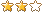[求助]客户编号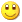Post By：2019/1/11 23:23:00 [只看该作者]

 老师有要麻烦你了。我想把下面的代码改成能像剪切图片中显示的格式一样。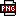此主题相关图片如下：image 3.png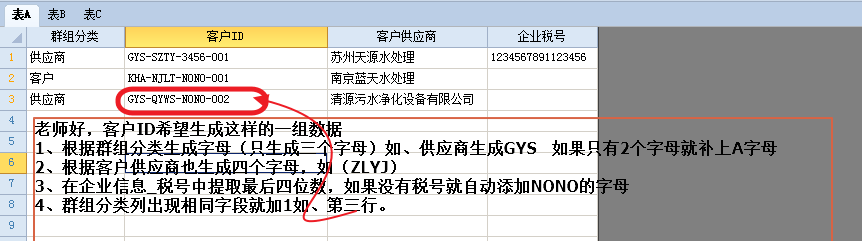Select e.DataCol.Name    Case "群组分类","客户供应商","企业信息_税号"        If e.DataRow.IsNull("群组分类") OrElse e.DataRow.Isnull("客户供应商") OrElse e.DataRow.Isnull("企业信息_税号") Then            e.DataRow("客户ID") = Nothing        Else            ' Dim lb As String = getpy(e.DataRow("商品名称")) & "-" & e.DataRow("型号") & "-" & e.DataRow("规格") & "-"            Dim lb As String = getpy(e.DataRow("群组分类"), True).toupper & "-" & e.DataRow("客户供应商") & "-" & e.DataRow("企业信息_税号") & "-"            Dim max As String = e.DataTable.Compute("Max(客户ID)","客户id like '" & lb & "%' And _Identify<> '" & e.DataRow("_Identify") & "'") '取得该类别的最大编号            Dim idx = 1            If max > "" Then                idx = cint(max.SubString(lb.length))+1            End If            e.DataRow("客户ID") = lb & format(idx, "00")        End IfEnd Select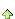2楼 | 信息 | 搜索 | 邮箱 | 主页 | UC加好友发短信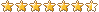Post By：2019/1/12 9:52:00 [只看该作者]

 Select e.DataCol.Name    Case "群组分类","客户供应商","企业信息_税号"        If e.DataRow.IsNull("群组分类") OrElse e.DataRow.Isnull("客户供应商") OrElse e.DataRow.Isnull("企业信息_税号") Then            e.DataRow("客户ID") = Nothing        Else            Dim xz As String = getpy(e.DataRow("群组分类"), True).toupper().PadRight(4,"A")            Dim gys As String = e.DataRow("客户供应商").toupper()            If gys.Length > 3 Then gys = gys.Substring(0,4)            Dim sh As String = e.DataRow("企业信息_税号")            If sh.Length > 3 Then sh = sh.SubString(sh.Length - 4)            Dim lb As String = xz & "-" & gys & "-" & sh & "-"            Dim max As String = e.DataTable.Compute("Max(客户ID)","客户id like '" & lb & "%' And _Identify <> " & e.DataRow("_Identify") ) '取得该类别的最大编号            Dim idx = 1            If max > "" Then                idx = cint(max.SubString(lb.length))+1            End If            e.DataRow("客户ID") = lb & format(idx, "000")        End IfEnd Select3楼 | 信息 | 搜索 | 邮箱 | 主页 | UC加好友发短信Post By：2019/1/12 18:39:00 [只看该作者]

首先谢谢老师此主题相关图片如下：image 2.png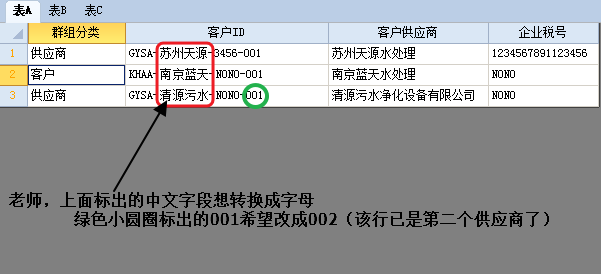下载信息  [文件大小：   下载次数： ]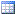点击浏览该文件:编号设置.table4楼 | 信息 | 搜索 | 邮箱 | 主页 | UC加好友发短信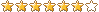Post By：2019/1/13 22:27:00 [只看该作者]

 Select e.DataCol.Name    Case "群组分类","客户供应商","企业税号"        If e.DataRow.IsNull("群组分类") OrElse e.DataRow.Isnull("客户供应商") OrElse e.DataRow.Isnull("企业税号") Then            e.DataRow("客户ID") = Nothing        Else            Dim xz As String = getpy(e.DataRow("群组分类"), True).toupper().PadRight(4,"A")            Dim gys As String = e.DataRow("客户供应商").toupper()            If gys.Length > 3 Then gys = gys.Substring(0,4)            Dim sh As String = e.DataRow("企业税号")            If sh.Length > 3 Then sh = sh.SubString(sh.Length - 4)            Dim lb As String = xz & "-" & getpinyin(gys,1).Toupper & "-" & sh & "-"            Dim max As String = e.DataTable.Compute("Max(客户ID)","群组分类 = '" & e.DataRow("群组分类") & "' And _Identify <> " & e.DataRow("_Identify") ) '取得该类别的最大编号            Dim idx = 1            If max > "" Then                idx = cint(max.SubString(lb.length))+1            End If            e.DataRow("客户ID") = lb & format(idx, "000")        End IfEnd Select5楼 | 信息 | 搜索 | 邮箱 | 主页 | UC加好友发短信Post By：2019/1/14 18:47:00 [只看该作者]此主题相关图片如下：image 2.png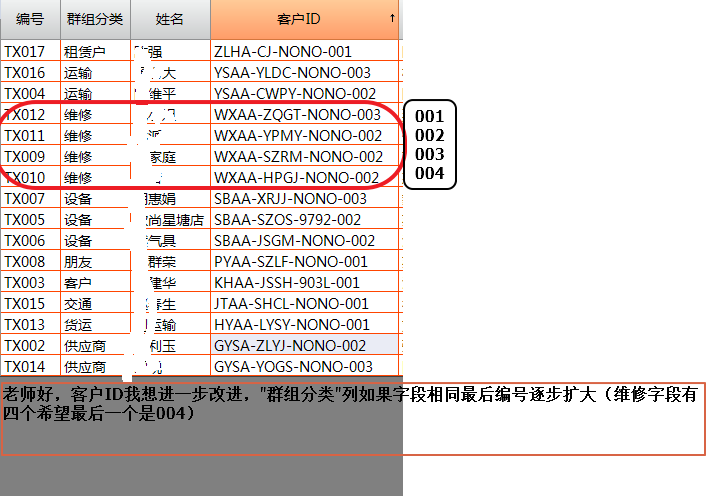6楼 | 信息 | 搜索 | 邮箱 | 主页 | UC加好友发短信Post By：2019/1/14 20:31:00 [只看该作者]

 Dim max As String = e.DataTable.Compute("Max(客户ID)","群组分类 = '" & e.DataRow("群组分类") & "' And _Identify <> " & e.DataRow("_Identify") ) 改为 Dim max As String = e.DataTable.Compute("Max(客户ID)","客户ID like '" & xz & "%' And _Identify <> " & e.DataRow("_Identify") )7楼 | 信息 | 搜索 | 邮箱 | 主页 | UC加好友发短信Post By：2019/1/14 21:05:00 [只看该作者]

 老师，每重置群组分类列客户ID列会扩大编号。8楼 | 信息 | 搜索 | 邮箱 | 主页 | UC加好友发短信Post By：2019/1/14 21:35:00 [只看该作者]

 Select e.DataCol.Name    Case "群组分类","客户供应商","企业税号"        If e.DataRow.IsNull("群组分类") OrElse e.DataRow.Isnull("客户供应商") OrElse e.DataRow.Isnull("企业税号") Then            e.DataRow("客户ID") = Nothing        Else            Dim xz As String = getpy(e.DataRow("群组分类"), True).toupper().PadRight(4,"A")            Dim gys As String = e.DataRow("客户供应商").toupper()            If gys.Length > 3 Then gys = gys.Substring(0,4)            Dim sh As String = e.DataRow("企业税号")            If sh.Length > 3 Then sh = sh.SubString(sh.Length - 4)            Dim lb As String = xz & "-" & getpinyin(gys,1).Toupper & "-" & sh & "-"            If e.DataRow("客户ID").StartsWith(lb) = False                Dim max As String = e.DataTable.SQLCompute("Max(Right(客户ID,3))","客户ID like '" & xz & "%' And [_Identify] <> " & e.DataRow("_Identify") ) '取得该类别的最大编号                Dim idx = 1                If max > "" Then                    idx = cint(max.SubString(max.length - 3))+1                End If                e.DataRow("客户ID") = lb & format(idx, "000")                e.DataRow.save            End If        End IfEnd Select9楼 | 信息 | 搜索 | 邮箱 | 主页 | UC加好友发短信Post By：2019/1/14 21:54:00 [只看该作者]

 老师，假如我把客户ID列的数据删除然后重置群组分类列此时客户ID列的数据还是会扩展。10楼 | 信息 | 搜索 | 邮箱 | 主页 | UC加好友发短信Post By：2019/1/14 22:00:00 [只看该作者]

 数据删除后先保存再重置列总数 11 1 2 下一页